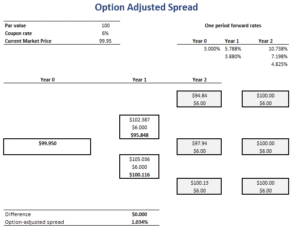The Option Adjusted Spread (OAS) is used to value risky bonds using backward induction. In particular, we use risk-free rates to value the risky bond. In that case, we will end up with a bond price that is too high. To correct for this, we add a constant spread to all the risk-free rates such that the bond price we obtain matches the market price of the risky bond. This value is the option adjusted spread.

On this page, we discuss the option adjusted spread for dummies. At the bottom of the page, we also include a OAS calculator which illustrates how to calculate the option adjusted spread in Excel.

There is no closed-form formula that we can use to calculate the OAS. Instead, however, we need to use Excel’s Solver to calculate the constant spread needed. Let’s go over the necessary steps to calculate the OAS.

1. First, we need to collect and/or calculate one-period forward rates for our interest rate tree.
2. Second, we construct a binomial interest rate tree to solve the option price using the one-period forward rates
3. Third, we determine the constant spread that should be added such that the bond price equals the observed bond price.

How to calculate the OAS? The steps above summarise the process. Still, to fully grasp the methodology, a numerical example is probably helpful. Thus, we have implemented the above steps using a simple numerical example. Also, we use the Solver to find the OAS needed to balance the stock price from the interest rate tree with the observed bond price.